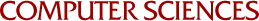## Quasilinear Parabolic Boundary Value Problems. Approximate Solutions and Error Bounds by Linear Programming

T-Yat Cheung
1971

Constrained minimization problems are formulated from a quasilinear parabolic boundary value problem (probably with nonlinear boundary conditions), making use of the latters (conditional) inverse-positive property. Approximate solutions and three error bounds can be obtained by solving these minimization problems by linear programming and discretization techniques. Numerical results are obtained using splines as basis functions.